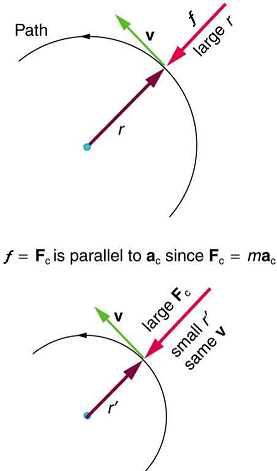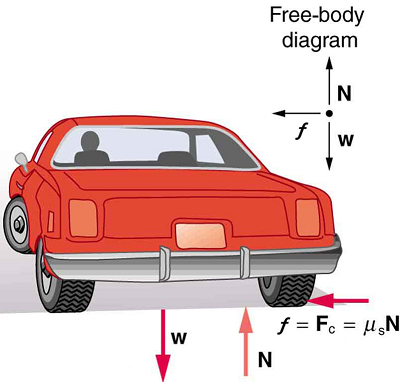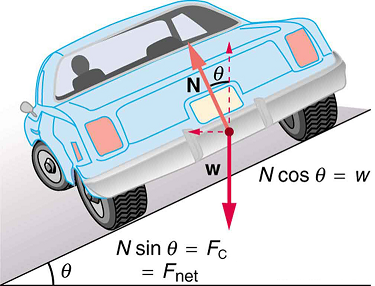$$\require{cancel}$$

# 6.4: Centripetal Force

•• Contributed by OpenStax
• General Physics at OpenStax CNX

Any force or combination of forces can cause a centripetal or radial acceleration. Just a few examples are the tension in the rope on a tether ball, the force of Earth’s gravity on the Moon, friction between roller skates and a rink floor, a banked roadway’s force on a car, and forces on the tube of a spinning centrifuge.

Any net force causing uniform circular motion is called a centripetal force. The direction of a centripetal force is toward the center of curvature, the same as the direction of centripetal acceleration. According to Newton’s second law of motion, net force is mass times acceleration: net $$F = ma$$. For uniform circular motion, the acceleration is the centripetal acceleration - $$a = a_c$$. Thus, the magnitude of centripetal force $$F_c$$ is $F_c = ma_c.$

By using the expressions for centripetal acceleration $$a_c$$ from $$a_c = \frac{v^2}{r}; \, a_c = r\omega^2$$, we get two expressions for the centripetal force $$F_c$$ in terms of mass, velocity, angular velocity, and radius of curvature:

$F_c = m \dfrac{v^2}{r}; \, F_c = mr\omega^2.$

You may use whichever expression for centripetal force is more convenient. Centripetal force $$F_c$$ is always perpendicular to the path and pointing to the center of curvature, because $$a_c$$ is perpendicular to the velocity and pointing to the center of curvature.Note that if you solve the first expression for $$r$$, you get $r = \dfrac{mv^2}{F_c}.$

This implies that for a given mass and velocity, a large centripetal force causes a small radius of curvature—that is, a tight curve.Figure $$\PageIndex{1}$$:The frictional force supplies the centripetal force and is numerically equal to it. Centripetal force is perpendicular to velocity and causes uniform circular motion. The larger the $$F_c$$, the smaller the radius of curvature $$r$$ and the sharper the curve. The second curve has the same $$v$$, but a larger $$F_c$$ produces a smaller $$r'$$.

Example $$\PageIndex{1}$$: What Coefficient of Friction Do Car Tires Need on a Flat Curve?

1. Calculate the centripetal force exerted on a 900 kg car that negotiates a 500 m radius curve at 25.0 m/s.
2. Assuming an unbanked curve, find the minimum static coefficient of friction, between the tires and the road, static friction being the reason that keeps the car from slipping (see Figure).

Strategy and Solution for (a)

We know that $$F_c = \frac{mv^2}{r}.$$ Thus, $F_c = \dfrac{mv^2}{r} = \dfrac{(900\, kg)(25.0 \, m/s)^2}{500 \, m)} = 1125 \, N. \nonumber$

Strategy for (b)

Figure shows the forces acting on the car on an unbanked (level ground) curve.Figure $$\PageIndex{2}$$: This car on level ground is moving away and turning to the left. The centripetal force causing the car to turn in a circular path is due to friction between the tires and the road. A minimum coefficient of friction is needed, or the car will move in a larger-radius curve and leave the roadway.

Friction is to the left, keeping the car from slipping, and because it is the only horizontal force acting on the car, the friction is the centripetal force in this case. We know that the maximum static friction (at which the tires roll but do not slip) is $$\mu_s N,$$ where $$\mu_s$$ is the static coefficient of friction and N is the normal force. The normal force equals the car’s weight on level ground,)so that $$N = mg$$. Thus the centripetal force in this situation is

$F_c = f = \mu_sN = \mu_s mg. \nonumber$

Now we have a relationship between centripetal force and the coefficient of friction. Using the first expression for $$F_c$$ from the equation

\begin{align*} F_c = m\dfrac{v^2}{r} \\[5pt] &= mr\omega^2 \end{align*}

$m\dfrac{v^2}{r} = \mu_smg. \nonumber$

We solve this for $$\mu_s$$, noting that mass cancels, and obtain

$\mu_s = \dfrac{v^2}{rg}. \nonumber$

Solution for (b)

Substituting the knowns,

$\mu_s = \dfrac{(25.0 \, m/s)^2}{(500 \, m)(9.80 \, m/s^2)} = 0.13. \nonumber$

(Because coefficients of friction are approximate, the answer is given to only two digits.)

Discussion

We could also solve part (a) using the first expression in

\begin{align*} F_c &= m\dfrac{v^2}{r} \\[4pt] &= mr\omega^2 \end{align*}

because $$m$$, $$v$$ and $$r$$ are given. The coefficient of friction found in part (b) is much smaller than is typically found between tires and roads. The car will still negotiate the curve if the coefficient is greater than 0.13, because static friction is a responsive force, being able to assume a value less than but no more than $$\mu_sN$$. A higher coefficient would also allow the car to negotiate the curve at a higher speed, but if the coefficient of friction is less, the safe speed would be less than 25 m/s. Note that mass cancels, implying that in this example, it does not matter how heavily loaded the car is to negotiate the turn. Mass cancels because friction is assumed proportional to the normal force, which in turn is proportional to mass. If the surface of the road were banked, the normal force would be less as will be discussed below.

Let us now consider banked curves, where the slope of the road helps you negotiate the curve. See Figure. The greater the angle $$\theta$$, the faster you can take the curve. Race tracks for bikes as well as cars, for example, often have steeply banked curves. In an “ideally banked curve,” the angle $$\theta$$ is such that you can negotiate the curve at a certain speed without the aid of friction between the tires and the road. We will derive an expression for $$\theta$$ for an ideally banked curve and consider an example related to it.

For ideal banking, the net external force equals the horizontal centripetal force in the absence of friction. The components of the normal force N in the horizontal and vertical directions must equal the centripetal force and the weight of the car, respectively. In cases in which forces are not parallel, it is most convenient to consider components along perpendicular axes—in this case, the vertical and horizontal directions.

Figure shows a free body diagram for a car on a frictionless banked curve. If the angle $$\theta$$ is ideal for the speed and radius, then the net external force will equal the necessary centripetal force. The only two external forces acting on the car are its weight $$w$$ and the normal force of the road $$N$$. (A frictionless surface can only exert a force perpendicular to the surface—that is, a normal force.) These two forces must add to give a net external force that is horizontal toward the center of curvature and has magnitude $$mv^2/r$$. Because this is the crucial force and it is horizontal, we use a coordinate system with vertical and horizontal axes. Only the normal force has a horizontal component, and so this must equal the centripetal force—that is,

$N\, \sin \, \theta = \dfrac{mv^2}{r}.$

Because the car does not leave the surface of the road, the net vertical force must be zero, meaning that the vertical components of the two external forces must be equal in magnitude and opposite in direction. From the figure, we see that the vertical component of the normal force is $$N\, cos \, \theta$$, and the only other vertical force is the car’s weight. These must be equal in magnitude; thus,

$N\, \cos \, \theta = mg.$

Now we can combine the last two equations to eliminate $$N$$ and get an expression for $$\theta$$, as desired. Solving the second equation for $$N = mg/(cos \, \theta)$$, and substituting this into the first yields

$mg\dfrac{\sin\, \theta}{\cos \, \theta} = \dfrac{mv^2}{r}$

$mg \, tan \, \theta = \dfrac{mv^2}{r}$

$\tan \, \theta = \dfrac{v^2}{rg}$

Taking the inverse tangent gives

$\theta = \tan^{-1} \left( \dfrac{v^2}{rg} \right) \, (ideally \, banked \, curve, \, no \, friction).$

This expression can be understood by considering how $$\theta$$ depends on $$v$$ and $$r$$. A large $$\theta$$ will be obtained for a large $$v$$ and a small $$r$$. That is, roads must be steeply banked for high speeds and sharp curves. Friction helps, because it allows you to take the curve at greater or lower speed than if the curve is frictionless. Note that $$\theta$$ does not depend on the mass of the vehicle.Figure $$\PageIndex{3}$$: The car on this banked curve is moving away and turning to the left.

Example $$\PageIndex{2}$$: What is the Ideal Speed to Take a Steeply Banked Tight Curve?

Curves on some test tracks and race courses, such as the Daytona International Speedway in Florida, are very steeply banked. This banking, with the aid of tire friction and very stable car configurations, allows the curves to be taken at very high speed. To illustrate, calculate the speed at which a 100 m radius curve banked at 65.0° should be driven if the road is frictionless.

Strategy

We first note that all terms in the expression for the ideal angle of a banked curve except for speed are known; thus, we need only rearrange it so that speed appears on the left-hand side and then substitute known quantities.

Solution

Starting with

$\tan \, \theta = \dfrac{v^2}{rg} \nonumber$

we get

$v = (rg \, tan \, \theta)^{\frac{1}{2}}. \nonumber$

Noting that tan 65.0º = 2.14, we obtain

\begin{align*} v &= [(100 \, m)(9.80 \, m/s^2)(2.14)]^{\frac{1}{2}} \\[4pt] &= 45.8 \, m/s \end{align*}

Discussion

This is just about 165 km/h, consistent with a very steeply banked and rather sharp curve. Tire friction enables a vehicle to take the curve at significantly higher speeds.

Calculations similar to those in the preceding examples can be performed for a host of interesting situations in which centripetal force is involved—a number of these are presented in this chapter’s Problems and Exercises.

TAKE-HOME EXPERIMENT

Ask a friend or relative to swing a golf club or a tennis racquet. Take appropriate measurements to estimate the centripetal acceleration of the end of the club or racquet. You may choose to do this in slow motion.

PHET EXPLORATIONS: GRAVITY AND ORBITS

Move the sun, earth, moon and space station to see how it affects their gravitational forces and orbital paths. Visualize the sizes and distances between different heavenly bodies, and turn off gravity to see what would happen without it!

# Summary

• Centripetal force $$F_c$$ is any force causing uniform circular motion. It is a “center-seeking” force that always points toward the center of rotation. It is perpendicular to linear velocity $$v$$ and has magnitude $F_c = ma_c \nonumber$ which can also be expressed as $F_c = \dfrac{v^2}{r} \nonumber$ or $F_c = mr\omega^2 \nonumber$

## Glossary

centripetal force
any net force causing uniform circular motion
ideal banking
the sloping of a curve in a road, where the angle of the slope allows the vehicle to negotiate the curve at a certain speed without the aid of friction between the tires and the road; the net external force on the vehicle equals the horizontal centripetal force in the absence of friction
ideal speed
the maximum safe speed at which a vehicle can turn on a curve without the aid of friction between the tire and the road
ideal angle
the angle at which a car can turn safely on a steep curve, which is in proportion to the ideal speed
banked curve
the curve in a road that is sloping in a manner that helps a vehicle negotiate the curve

## Contributors

• Paul Peter Urone (Professor Emeritus at California State University, Sacramento) and Roger Hinrichs (State University of New York, College at Oswego) with Contributing Authors: Kim Dirks (University of Auckland) and Manjula Sharma (University of Sydney). This work is licensed by OpenStax University Physics under a Creative Commons Attribution License (by 4.0).